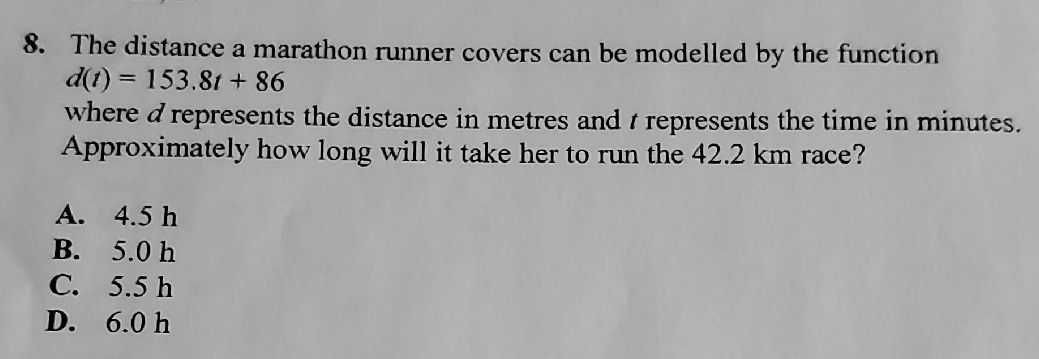### ¿Todavía tienes preguntas de matemáticas?

Pregunte a nuestros tutores expertos
Algebra
Pregunta8. The distance a marathon runner covers can be modelled by the function

$$d ( t ) = 153.8 t + 86$$ where $$d$$ represents the distance in metres and $$t$$ represents the time in minutes. Approximately how long will it take her to run the $$42.2 km$$ race?

A. $$4.5 h$$ B. $$5.0 h$$ C. $$5.5 h$$ D. $$6.0 h$$